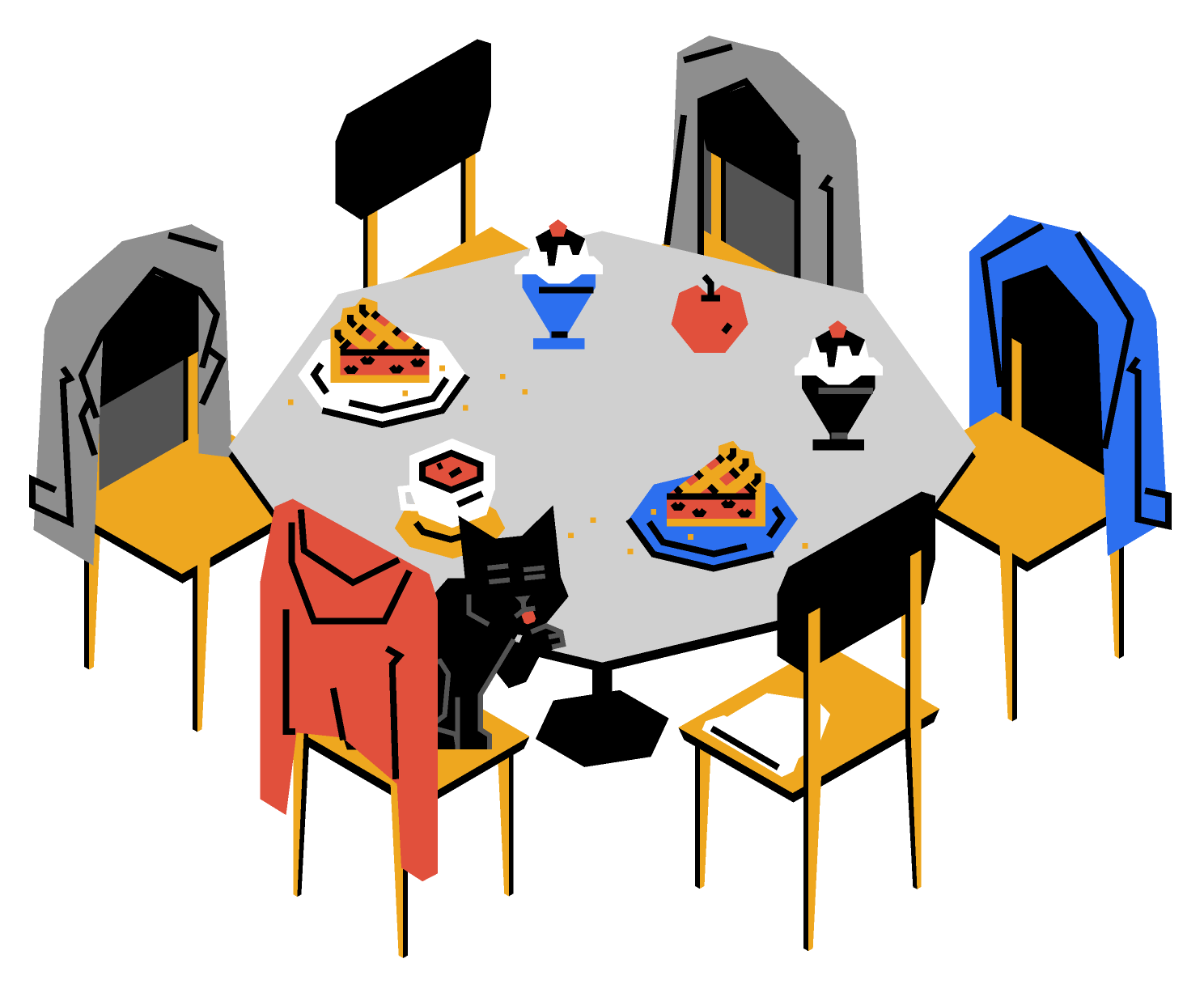Back

## Wrong Seats

You open a book in English and pick some words at random. How many words would you have to read to ensure that at least two of them start with the same letter?

If you read just $2$ words, they can easily start with different letters, say A and B. Adding an extra word doesn't help much, because it could start with C, for example. It's possible, albeit unlikely, that even $26$ words start with different letters from A to Z. However, if you add $1$ more word, its first letter must have been already used simply because the English alphabet contains fewer than $27$ letters.

This is a direct consequence of a seemingly obvious but very powerful theorem, the pigeonhole principle:

If $n$ items are put into $m$ containers, where $n > m > 0,$ then at least one container must contain more than one item.

In this case, we put $27$ "items" (words) into $26$ "containers" (letters), so one letter had to be assigned two words.

We'll take a look at one more application, this time in number theory. Let's choose at random $51$ distinct integers between $1$ and $100.$ The pigeonhole principle tells us that at least two of our integers are consecutive. Why?

Suppose we start at $1$ and pair off consecutive integers so we have $50$ sets $\{1, 2\}, \{3, 4\}, \dots, \{99, 100\}.$ This gives us $50$ "containers" to put our "items" into. Since we pick $51$ numbers ("items"), at least one of our "containers" must hold two numbers, which are a consecutive pair.

Now you're ready to tackle today's challenge!

# Today's Challenge

A round table at a party has six chairs labeled with guest names. Today, all six guests neglected the labels and sat in the wrong seats.The party manager, aghast, politely asked everyone to move to their assigned seats. The guests then each moved the same number of seats clockwise around the table until the number of correctly seated guests was maximized.

Considering all possible configurations of the wrong seating in the beginning, what is the smallest possible number of guests sitting in the correct chairs after this re-seating process? $\\[-0.5em]$×